# 2.1 Applications of Three-Dimensional Device Simulation

Three-dimensional device simulation is mandatory for investigations on real three-dimensional structures because these structures cannot be described by two-dimensional cuts. As an example, Fig. 2.1 shows the three-dimensional structure of a Lateral Trench LDMOSFET. The figure shows the device at breakdown with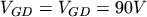. The gate is built by a trench whereas the channel forms at the sidewall of the trench gate vertically in the device. Due to the super-junction concept the drift area is fully depleted at breakdown. The potential is uniformly distributed to account for the Reduced Surface Field (RESURF) effect.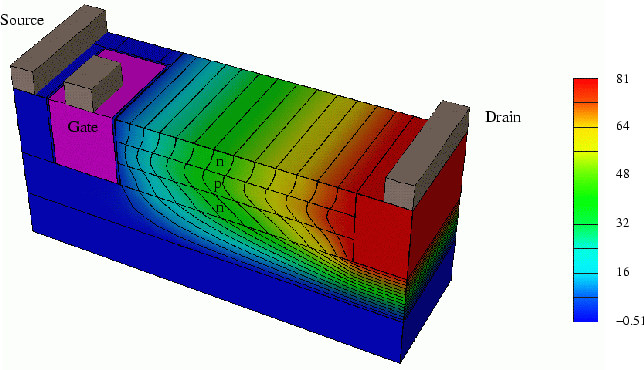Furthermore, three-dimensional simulations are mandatory when feature sizes become so small that the three-dimensional shape of geometries cannot be neglected. Thus two-dimensional simulations are no more reliable. A frequently cited example is the narrow channel effect of MOS transistors which are normally simulated in two-dimensional cuts. When the width of the channel becomes comparable to its length, the current path becomes clearly three-dimensional.

Another more obvious example is the temperature profile in real devices. Two-dimensional simulations cannot predict temperature profiles very well, because they do not take all cooling plates and directions of the heat flow into account. As an example Fig. 2.2 shows the geometry of a body contacted SOI MOS transistor. The gate is T-shaped and its length is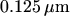and the width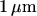. The gate oxide thickness is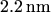. The transistor is surrounded by a rectangular silicon area of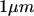width which has been used here only to increase the cooling area and therefore to make the resulting temperature profile more visible.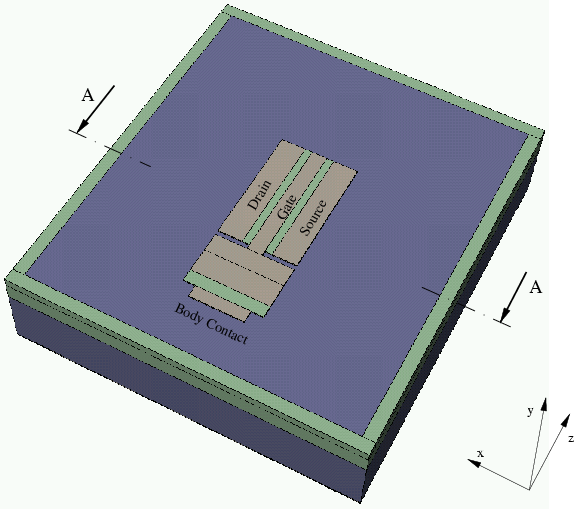Fig. 2.3 shows the result of a three-dimensional simulation. At the thermal contacts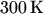was applied. The temperature profile is clearly three-dimensional contrary to the assumptions made for two-dimensional simulations. At the end of the gate in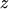-direction the temperature is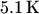lower than in the middle.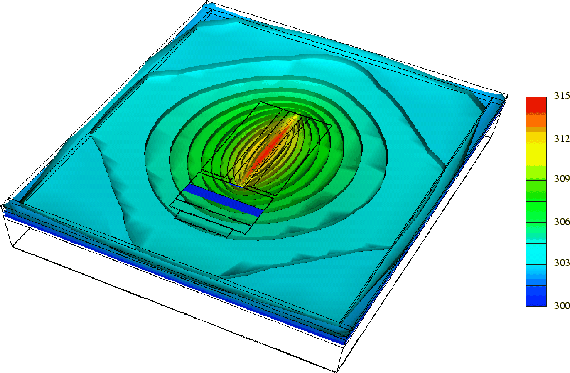Fig. 2.4(a) shows the result of a two-dimensional simulation performed for the cut A-A depicted in Fig. 2.2. As a comparison, in Fig. 2.4(b) the same cut A-A is shown for the three-dimensional simulation of Fig. 2.3. As expected, the highest temperature appears in the channel below the gate and decreases with increasing lateral distance to the channel. Because the thermal resistance of SiO2 is much higher than that of silicon, a high temperature range is supplied by the gate.

Figure 2.4: Temperature profiles [K] in cut A-A of a two- and a three-dimensional simulation.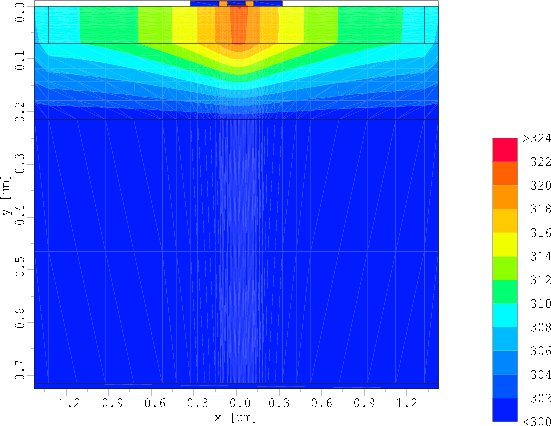(a)  Two-dimensional simulation.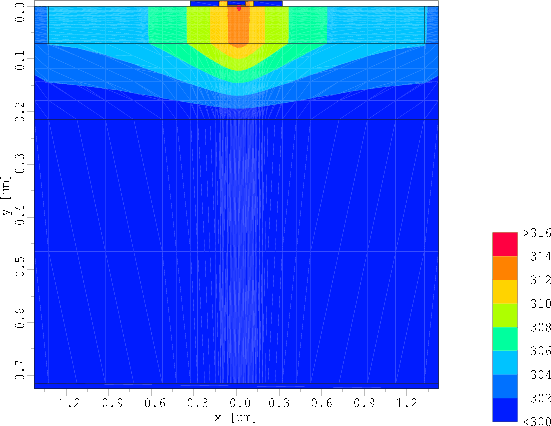(b)  Three-dimensional simulation.

To clearly stress out this result the temperature at the surface of the silicon segment of both the two-dimensional simulation ( Fig. 2.4(a)) and the cut of the three-dimensional result (Fig. 2.4(b)) is shown in Fig. 2.5. It can be clearly seen, that the two-dimensional simulation predicts a much higher temperature compared to the three-dimensional one. The maximal temperature in the channel below the gate is calculated asfor the two-dimensional case and as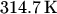for the three-dimensional case. Therefore, the error of the temperature increase due to self heating made by the two-dimensional simulation is about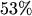below the gate and about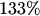at the side.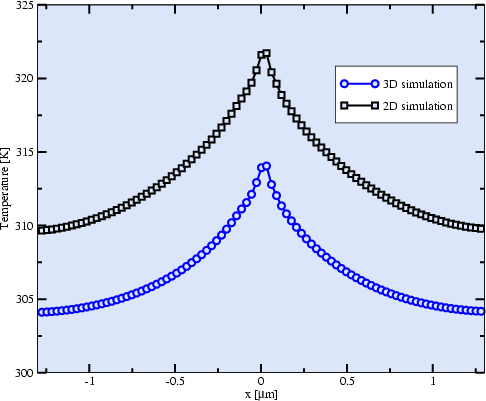Fig. 2.6 and Fig. 2.7 show the temperature profile of a body contacted SOI MOS transistor with a gate width of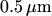and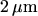, respectively. Both figures show a three-dimensional shape of the temperature inside the device. But from these figures one can clearly see the increasing importance of three-dimensional simulations with decreasing feature sizes of the devices. Two-dimensional simulations are no more accurate enough, not even for long devices.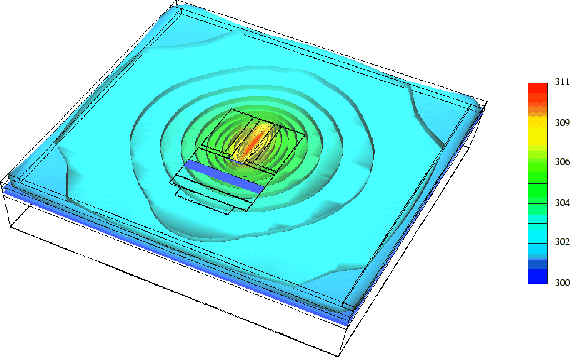Robert Klima 2003-02-06